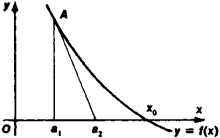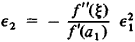# Newton's method

(redirected from Newton-Rhapson algorithm)

## Newton's method

[′nüt·ənz ‚meth·əd]
(mathematics)
A technique to approximate the roots of an equation by the methods of the calculus.
McGraw-Hill Dictionary of Scientific & Technical Terms, 6E, Copyright © 2003 by The McGraw-Hill Companies, Inc.
The following article is from The Great Soviet Encyclopedia (1979). It might be outdated or ideologically biased.

## Newton’s Method

a method of approximating a root x0 of the equation f(x) = 0; also called the method of tangents. In Newton’s method, the initial (“first”) approximation x = a1 is used to find a second, more accurate, approximation by drawing the tangent to the graph of y = f(x) at the point A[a1, f(a1)] up to the intersection of the tangent with the Ox-axis (see Figure 1). The point of intersection is x = a1f(a1)/f’(a1) and is adopted as the new value a2 of the root. By repeating this process as necessary, we can obtain increasingly accurate approximations a2, a3, … of the root x0 provided that the derivative f’(x) is monotonic and preserves its sign on the segment containing x0.The error ε2 = x0a2 of the new value a2 is related to the old error ε1 = x0a1 by the formulawhere f”(ξ) is the value of the second derivative of the function f(x) at some point ξ that lies between x0 and a 1. It is sometimes recommended that Newton’s method be used in conjunction with some other method, such as linear interpolation. Newton’s method allows generalizations, which makes it possible to use the method for solving equations f(x) = 0 in normed spaces, where F is an operator in such a space, in particular, for solving systems of equations and functional equations. This method was developed by I. Newton in 1669.